NCERT Solutions - Can we Share?

# Can we Share? NCERT Solutions - Mathematics for Class 3: NCERT

Page No. 159

Q.1.There are__________caterpillars. They are in__________groups. There are___________caterpillars in each group.
Ans.
There are 21 caterpillars.
They are in 3 groups.
There are 7 caterpillars in each group.

Q.2.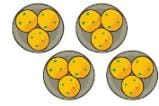There are__________laddoos. They are in__________groups. There are__________laddoos in each group.
Ans.
They are in 4 groups.
There are 3 laddoos in each group.

Page No. 160

Q.1.
Draw 18 stars. Put them into 2 equal groups.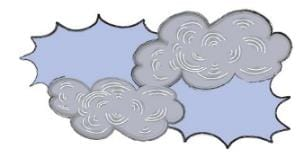There are ________ stars in each group.
Ans. There are 9 stars in each group.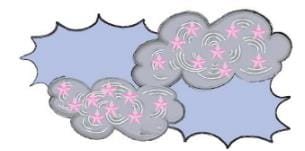Q.2.
Draw 18 beads. Put them into 3 equal groups.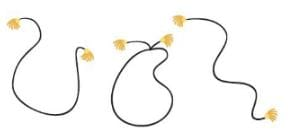There are ________ beads in each group.
Ans. There are 6 beads in each group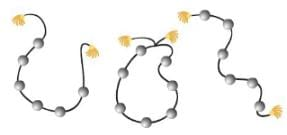Page No. 162

Q.1. Now draw the jalebis on the plates below, so that each plate has the same number of jalebis.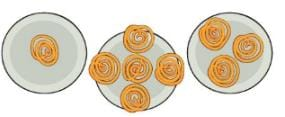How many jalebis are there altogether? _________How many jalebis are there in each plate? _________Discuss in the class how you found the answer.
Ans.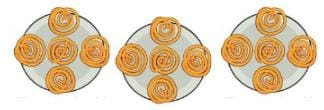There are 15 jalebis all together. There are 5 jalebis in each plate. Maximum number of jalebis in a plate is 5. So, plate A should have 4 more jalebis, and plate C should have 2 more jalebi.

Page No. 164

Q.1. If there are 60 bananas and two monkeys, how many will each monkey get? _________bananas. What if there are 600 bananas and two monkeys?
Ans. Number of bananas = 60
Number of monkeys = 2
Number of bananas each monkey gets =60÷2 = 30
Each monkey gets 30 bananas.
Number of bananas = 600
Number of monkeys = 2
Banana each monkey gets = 600÷2 = 300
Each monkey gets 300 bananas.

Q.2. Five friends found 10 five-rupee coins on the ground.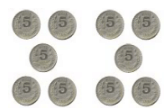They shared them equally. Each friend got ten rupees. 50 ÷ 5 = 10 If there are 16 ten-rupee notes and four friends to share, then 16 ÷ 4 =________and 4 × 10 = 40 so each friend gets________rupees. Five friends found Rs 100. If they share it equally, how much will each get?________Ans. 16 ÷ 4 = 4 and 4 × 10 = 40 So, each friend gets 40 rupees. 100 ÷ 5 = 20 So, each friend will get 20 rupees.

Q.3. Hari Prashad has 30 metres of rope. He distributes it equally among his three children. Each child gets_________ metres of rope. If there is 36 metres of rope, how much of rope will each child get?_________ And if there is 60 metres of rope, how much will each child get ___________.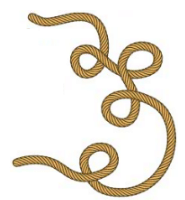Ans. Length of the rope = 30 metres
Number of children getting the rope = 3
Length of the rope each child gets    = 30 metres ÷3 = 10 metres
Each child will get 10 metres of the rope.
Length of the rope = 36 metres
Number of children getting the rope = 3
Length of the rope each child gets = 36 metres ÷3 = 12 metres
If there is 36 metres of rope, then each child will get 12 metres of rope.
Length of the rope = 60 metres
Number of children getting the rope = 3
Length of the rope each child gets = 60 metres ÷3 = 20 metres
If there is 60 metres of rope, then each child will get 20 metres of rope.

Page No. 167

Q.1. Minku puts her 15 laddoos equally into 5 boxes.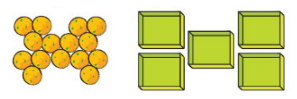(i) How many laddoos will there be in each box? There will be__________laddoos in each box. 15 ÷ 5 =_________
(ii) If she uses only 3 boxes, how many laddoos will there be in each box?There will be__________laddoos in each box. ________÷ 3 =________

Ans.
(i)  There are 15 laddoos, and 5 boxes are to be filled with these laddoos. So, number of laddoos in each box = 15 ÷ 5 = 3 There will be 3 laddoos in each box.
(ii) If she uses only 3 boxes. Then laddoos in each box = 15 ÷ 3 = 5.
There will be 5 laddoos in each box.

Q.2. Share 25 bananas among 5 monkeys. How many bananas for each monkey?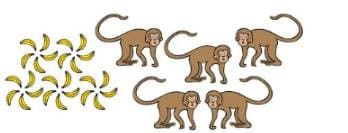______ ÷ 5 = ______ Each monkey has _________ bananas.
Ans. 25 ÷ 5 = 5
Each monkey has 5 bananas.

Page No. 168

Q.1. Share 12 balloons among 3 boys. How many balloons for each boy?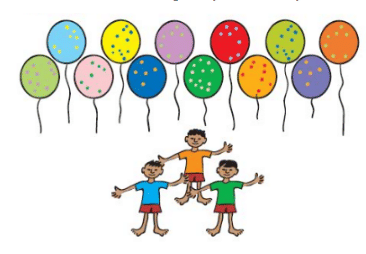_______ ÷ _______ = _______ Each boy has ________ balloons.
Ans. 12 ÷ 3 = 4
Each boy has 4 balloons.

Q.2. There are 21 candles. Put them equally in 3 boxes. How many candles are there in each box?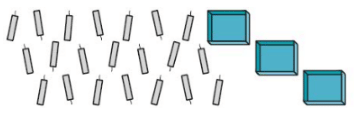Ans. 21 candles are to be put equally in 3 boxes. Number of candles in each box = 21 ÷ 3 = 7 So, there are 7 candles in each box.

Page No. 169

Q.1. There are 18 socks. How many girls can wear these socks?Ans. There are 18 socks. Each girl can wear 2 socks. Number of girls = 18÷2 = 9 So, 9 girls can wear 18 socks.

Q.2. Raj has 36 minutes to make rotis. One roti takes 3 minutes. How many rotis can he make in this time? He can make_________rotis.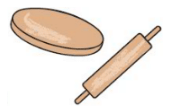Ans. Raj has only 36 minutes to make rotis. Each roti takes 3 minutes to prepare. Number of rotis he can make = 36÷3 = 12 He can make 12 rotis.

Q.3. These are 24 footmarks of goats. So how many goats were there?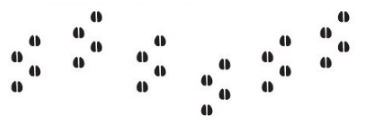Ans. Number of footmarks of goats = 24 Each goat has 4 legs. So number of goats = 24÷4 = 6 There were 6 goats.

Page No. 170

Q.1. Some girls are playing a game with both their hands. The girls who are playing have 60 fingers altogether. How many girls are playing this game?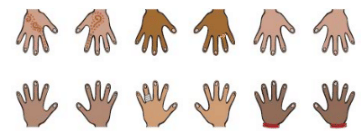Ans. Number of fingers of all the girls = 60 Each girl has 10 fingers. Number of girls  = 60÷10 = 6 6 girls are playing the game.

Q.2. Lakshmi has 27 kg potatoes to sell. Three men came and bought equal amounts of potatoes. Each man bought __________ kg of potatoes.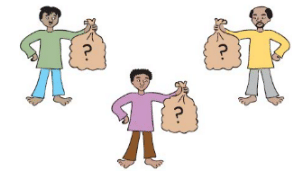Ans. Amount of potatoes to sell = 27 kg
Number of men who bought potatoes = 3
Amount of potatoes each of them bought = 27 kg ÷ 3 kg= 9 kg
Each man bought 9 kg of potatoes.

Page No. 171

Q.1. Jumpy Animals A frog jumps 2 steps at a time. A squirrel jumps 3 steps. A rabbit jumps 5 steps. A horse jumps 15 steps. A kangaroo jumps 30 steps. Use the path on the next page to find out:
1. In how many jumps will the frog reach 30? 30 ÷ 2 = _________
2. In how many jumps will the squirrel reach 27? 27 ÷ 3 = _________
3. Which number will the kangaroo reach in two jumps?
4. Who all will meet at the number 15? ________ , _______ , ________
5. Will the rabbit ever be at the number 18?________
6. How many jumps of the rabbit equal one jump of the horse? ________
7. How many jumps of the horse equals two jumps of the kangaroo?
8. Which is the smallest number where the frog and the squirrel will meet?
Ans.
1. Number of jumps frog takes to reach 30 = 30 ÷ 2 = 15 jumps Frog will take 15 jumps to reach 30.
2. Number of jumps squirrel takes to reach 27 = 27 ÷ 3 = 9 jumps Squirrel will take 9 jumps to reach 27.
3. Kangaroo takes 30 steps in one jump. It takes two jumps in total. So, in 2 jumps kangaroo will reach at number = 30×2 = 60 Kangaroo will reach at number 60 in two jumps.
4. Number 15 comes in the table of 3, 5 and 15. Squirrel will reach at 15 because 3×5 = 15 Rabbit will reach at 15 because 5×3 = 15 Horse will reach at 15 because 15×1 = 15 So, squirrel, rabbit, and horse will meet at number 15.
5. Rabbit takes five steps in one jump. In 2 jumps, rabbit will reach at number = 2×5 = 10 In 3 jumps, rabbit will reach at number = 3×5 = 15 In 4 jumps, rabbit will reach at number = 4×5 = 20 We can see that rabbit will not reach at number 18 in any of his jumps.
6. Horse takes 15 steps in one jump. Rabbit takes 5 steps in one jump. Steps covered by rabbit in 3 jumps = 3×5 = 15
Thus, horse takes 3 jumps to cover 15 steps. So, three jumps of rabbit is equal to one jump of the horse.
7. Kangaroo takes 30 steps in one jump. Steps covered by kangaroo in two jumps = 2×30 = 60 Horse takes 15 steps in one jump. Number of jumps taken by horse to cover 60 steps = 60÷15 = 4 Horse takes 4 jumps to cover 60 steps.
So, four jumps of the horse is equal to two jumps of the kangaroo.
8. Frog takes two steps in one jump. Frog takes steps 2, 4, 6, 8 and so on. Squirrel takes three steps in one jump. Squirrel takes steps, 3, 6, 12, 15 and so on. Number 6 is the common step of both frog and squirrel.
So, the smallest number where frog and squirrel will meet is 6.

Page No. 173

Q.1. Divide into groups of 2 using 2 times table.
i. 18 ÷ 2 = 9 Hint: 2 × 9 = 18
ii. 18 ÷ 9 = 2
iii. 16 ÷ 2 = __
iv. 20 ÷ 2 = __
v. __ ÷ 2 = 7
vi. __ ÷ 2 = 10
vii. 8 ÷ = 4
viii. __ ÷ 2 = 5
Ans.
i. 18 ÷ 2 = 9 Hint: 2 × 9 = 18
ii. 18 ÷ 9 = 2 Hint: 9 × 2 = 18
iii. 16 ÷ 2 = 8 Hint: 2 × 8 = 16
iv. 20 ÷ 2 = 10 Hint: 2 × 10 = 20
v. 14 ÷ 2 = 7 Hint: 2 × 7 = 14
vi. 20 ÷ 2 = 10 Hint: 2 × 10 = 20
vii. 8 ÷ 2 = 4 Hint: 2 × 4 = 8
viii. 10 ÷ 2 = 5 Hint: 2 × 5 = 10

Q.2. Divide into groups of 5 using 5 times table.
i. 10 ÷ 5 = ___ Hint: 5 × 2 = ?
ii. 20 ÷ ___ = 4
iii. 15 ÷ 5 = ___
iv. 40 ÷ ___8
v. 20 ÷ 5 = ___
vi. ___  ÷ 5 = 6
vii. 25 ÷ 5 = ___
viii.
___ ÷ 5 = 3
ix.
35 ÷ 5 = ___
x. ___  ÷ 5 = 2

Ans.
i. 10 ÷ 5 = 2 Hint: 5 × 2 = 10
ii. 20 ÷ = 4 Hint: 5 × 4 = 20
iii. 15 ÷ 5 = 3 Hint: 5 × 3 = 15
iv. 40 ÷ 5 = 8 Hint: 5 × 8 = 40
v. 20 ÷ 5 = 4 Hint: 5 × 4 = 20
vi. 30 ÷ 5 = 6 Hint: 5 × 6 = 30
vii. 25 ÷ 5 = 5 Hint: 5 × 5 = 25
viii. 15 ÷ 5 = 3 Hint: 5 × 3 = 15
ix. 35 ÷ 5 = 7 Hint: 5 × 7 = 35
x. 10 ÷ 5 = 2 Hint: 5 × 2 = 10

Page No. 174

Q.1. Try these.
i. 4 ÷ __ = 2
ii. 14 ÷ 7 = __
iii. 6 ÷ 3 = __
iv. __ ÷ 2 = 7
v. __ ÷ 2 = 3
vi. 15 ÷ 3 = __
vii. 8 ÷ 4 = __
viii. 15 ÷ 5 = __
ix. 8 ÷ __ = 4
x. __÷ 2 = 8
xi. 9 ÷ 3 = __
xii. 18 ÷ 9 = __
xiii. __ ÷ 2 = 5
xiv. 20 ÷ 5 = __
xv. 12 ÷ 4 = __
xvi.
20 ÷ 4 = __
xvii. 12 ÷ __ = 2

Ans.
i. 4 ÷ 2 = 2 Hint: 2 ×2 = 4
ii. 14 ÷ 7 = 2 Hint: 7 ×2 = 14
iii. 6 ÷ 3 = 2 Hint: 3 ×2 = 6
iv. 14 ÷ 2 = 7 Hint: 2 × 7 = 14
v. 6 ÷ 2 = 2 Hint: 2 × 3 = 6
vi. 15 ÷ 3 = 5 Hint: 3 × 5 = 15
vii. 8 ÷ 4 = 2 Hint: 4 × 2 = 8
viii. 15 ÷ 5 = 3 Hint: 5 × 3 = 15
ix. 8 ÷ 2 = 4 Hint: 2 × 4 = 8
x. 16 ÷ 2 = 8 Hint: 2 × 8 = 16
xi. 9 ÷ 3 = 3 Hint: 3 ×3 = 9
xii. 18 ÷ 9 = 2 Hint: 9 ×2 = 18
xiii. 10 ÷ 2 = 5 Hint: 2 × 5 = 10
xiv. 20 ÷ 4 = 5 Hint: 5 × 4 = 20
xv. 12 ÷ 4 = 3 Hint: 4 ×3 = 12
xvi. 20 ÷ 4 = 5 Hint: 4 × 5 = 20
xvii. 12 ÷ 6 = 2 Hint: 6 × 2 = 12

The document Can we Share? NCERT Solutions | Mathematics for Class 3: NCERT is a part of the Class 3 Course Mathematics for Class 3: NCERT.
All you need of Class 3 at this link: Class 3

## Mathematics for Class 3: NCERT

49 videos|119 docs|58 tests

## FAQs on Can we Share? NCERT Solutions - Mathematics for Class 3: NCERT

 1. What are NCERT solutions?Ans. NCERT solutions refer to the detailed answers to the questions present in NCERT textbooks. These solutions are designed to help students understand the concepts and solve problems effectively. They provide step-by-step explanations and solutions for various subjects and are widely used by students preparing for exams.
 2. Why are NCERT solutions important for exam preparation?Ans. NCERT solutions are important for exam preparation because they provide a comprehensive understanding of the topics covered in the NCERT textbooks. By referring to these solutions, students can clarify their doubts, practice different types of questions, and improve their problem-solving skills. Moreover, many exam questions are directly or indirectly based on NCERT textbooks, making these solutions crucial for scoring well in exams.
 3. Are NCERT solutions available for all subjects and classes?Ans. Yes, NCERT solutions are available for all major subjects taught in schools, including Mathematics, Science, Social Science, English, Hindi, and more. These solutions are available for different classes, ranging from Class 1 to Class 12. Students can easily find NCERT solutions online or in bookstores to aid their exam preparation.
 4. Where can I find reliable NCERT solutions for exam preparation?Ans. There are several reliable sources where you can find NCERT solutions for exam preparation. The official website of NCERT provides free access to these solutions for all subjects and classes. Additionally, there are many educational websites, online platforms, and mobile applications that offer comprehensive and accurate NCERT solutions. It is advisable to choose reputable sources and cross-check the solutions to ensure their reliability.
 5. How can NCERT solutions help in improving exam scores?Ans. NCERT solutions provide a strong foundation for exam preparation. By understanding the concepts and solving problems with the help of these solutions, students can enhance their knowledge and problem-solving abilities. The step-by-step explanations in these solutions enable students to grasp the underlying concepts better and tackle exam questions effectively. Regular practice using NCERT solutions can boost confidence, improve accuracy, and ultimately lead to higher exam scores.

## Mathematics for Class 3: NCERT

49 videos|119 docs|58 testsExplore Courses for Class 3 examSignup to see your scores go up within 7 days! Learn & Practice with 1000+ FREE Notes, Videos & Tests.
10M+ students study on EduRev
Track your progress, build streaks, highlight & save important lessons and more!
Related Searches

,

,

,

,

,

,

,

,

,

,

,

,

,

,

,

,

,

,

,

,

,

;